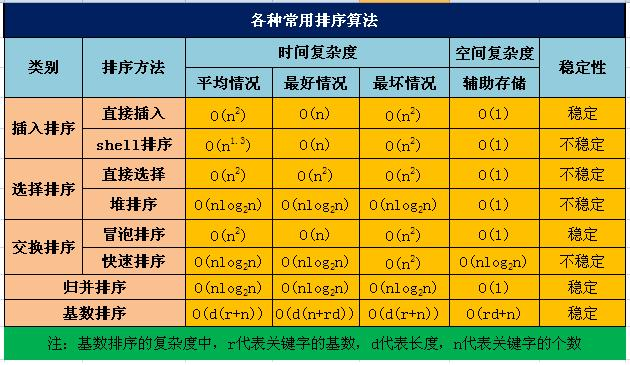# 数据结构中的参见排序算法的实现，以及时间复杂度和稳定性的分析（1）1.直接插入排序：直接插入排序是每次将要插入的数据与已排序的序列从后向前进行比较，如果已排序元素小于需要插入的数据，那么交换两者的位置，一直到达已排序序列头部为止。

#include <iostream>

using namespace std;

int main()
{
int s[]={10,9,8,7,6,5,4,3,2,1};

for(int i=0;i<10;i++)
{
int j=i+1;
while(s[j]<s[j-1]&&j>=1&&j<10)
{
int temp=s[j];
s[j]=s[j-1];
s[j-1]=temp;
j--;
}
}

for(int i=0;i<10;i++)
{
cout<<s[i]<<" ";
}
cout<<endl;
return 0;
}

Shell排序：Shell排序可以理解为是分组的直接插入排序，需要设置一个步长step（初始值为n/2），按照步长将序列分组，每组内部进行直接插入排序。

希尔排序的时间性能优于直接插入排序的原因：
①当文件初态基本有序时直接插入排序所需的比较和移动次数均较少。
②当n值较小时，n和n^2的差别也较小，即直接插入排序的最好时间复杂度O(n）和最坏时间复杂度0(n^2）差别不大。
③在希尔排序开始时增量较大，分组较多，每组的记录数目少，故各组内直接插入较快，后来增量di逐渐缩小，分组数逐渐减少，而各组的记录数目逐渐增多，但由于已经按di-1作为距离排过序，使文件较接近于有序状态，所以新的一趟排序过程也较快。

#include <iostream>

using namespace std;

int main()
{
int s[]={10,9,8,7,6,5,4,3,2,1};
int gap=10/2;
while(gap>=1)
{
for(int i=0;i<10;i++)
{
int j=i+gap;
while(j>=gap && j<10 && s[j]<s[j-gap])
{
int temp=s[j];
s[j]=s[j-gap];
s[j-gap]=temp;
j=j-gap;
}
}
gap=gap/2;
}
for(int i=0;i<10;i++)
{
cout<<s[i]<<" ";
}
cout<<endl;
return 0;
}

posted @ 2019-08-28 22:02 张杨 阅读(...) 评论(...) 编辑 收藏